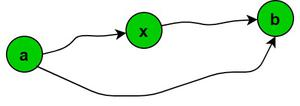Related Articles

# Shortest Path Properties

• Last Updated : 06 Jul, 2021

The shortest path problem is the problem of finding a path between two vertices (or nodes) in a graph such that the sum of the weights of its constituent edges is minimized. The shortest path between any two nodes of the graph can be founded using many algorithms, such as Dijkstra’s algorithm, Bellman-Ford algorithm, Floyd Warshall algorithm. There are some properties of finding the shortest paths based on which the algorithm to find the shortest path works:

1. Optimal Substructure Property
• All the sub-paths of the shortest path must also be the shortest paths.
• If there exists the shortest path length between two nodes U and V, then greedily choosing the edge with the minimum length between V to S will give the shortest path length between U and S.
• All the algorithms listed above work based on this property.
• For example, let P1 be a sub-path from (X → Y) of the shortest path (S →X →Y → V) of graph G. And let P2 be any other path (X Y) in graph G. Then, the cost of P1 must be less than or equal to the cost of P2. Otherwise, the path (S →X →Y → V) will not be the shortest path between nodes S and V.Graph G

2. Triangle Inequality
• Let d(a, b) be the length of the shortest path from a to b in graph G1. Then,
• d(a, b) ≤ d(a, x) + d(x, b)Graph G1

Attention reader! Don’t stop learning now. Get hold of all the important DSA concepts with the DSA Self Paced Course at a student-friendly price and become industry ready.  To complete your preparation from learning a language to DS Algo and many more,  please refer Complete Interview Preparation Course.

In case you wish to attend live classes with experts, please refer DSA Live Classes for Working Professionals and Competitive Programming Live for Students.

My Personal Notes arrow_drop_up